Question

# Continuous random variables X and Y have joint pdf fX,Y (x, y) = x +(1/y^2) defined...

Continuous random variables X and Y have joint pdf fX,Y (x, y) = x +(1/y^2) defined on the rectangular region 0 ≤ X ≤ 1 and 1 ≤ Y ≤ 2. What is the probability that X + Y ≤ 2?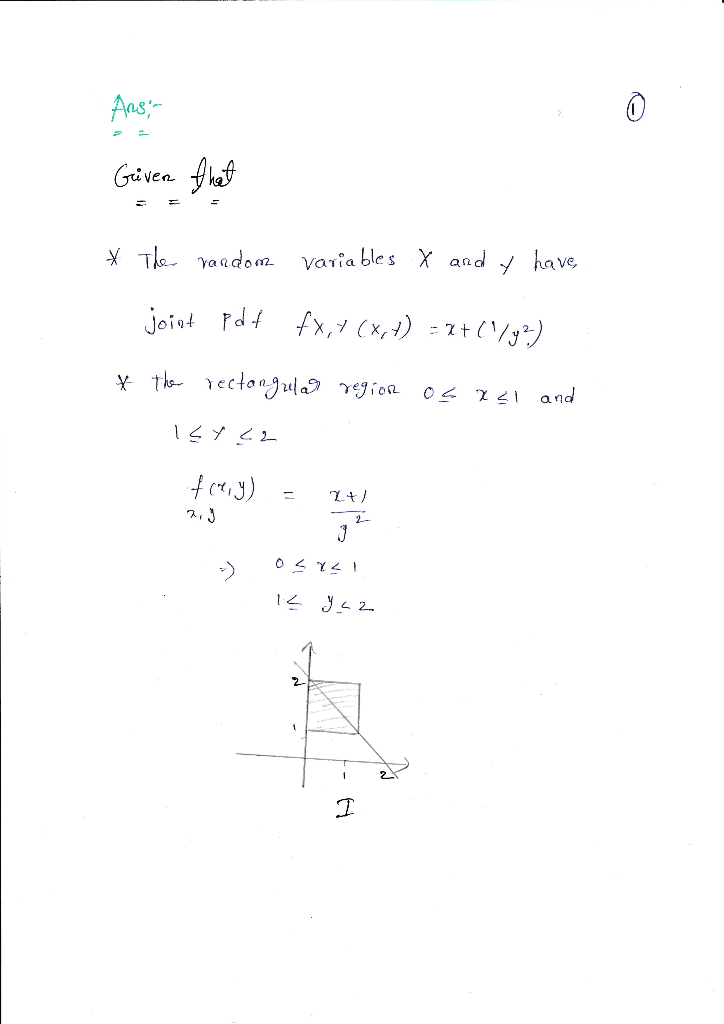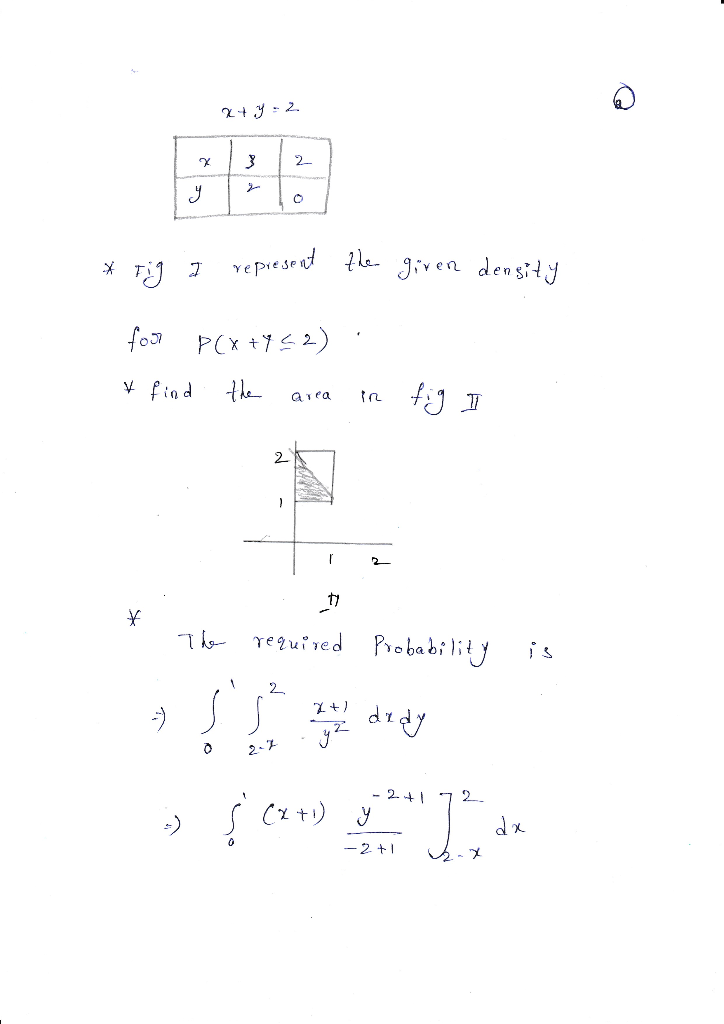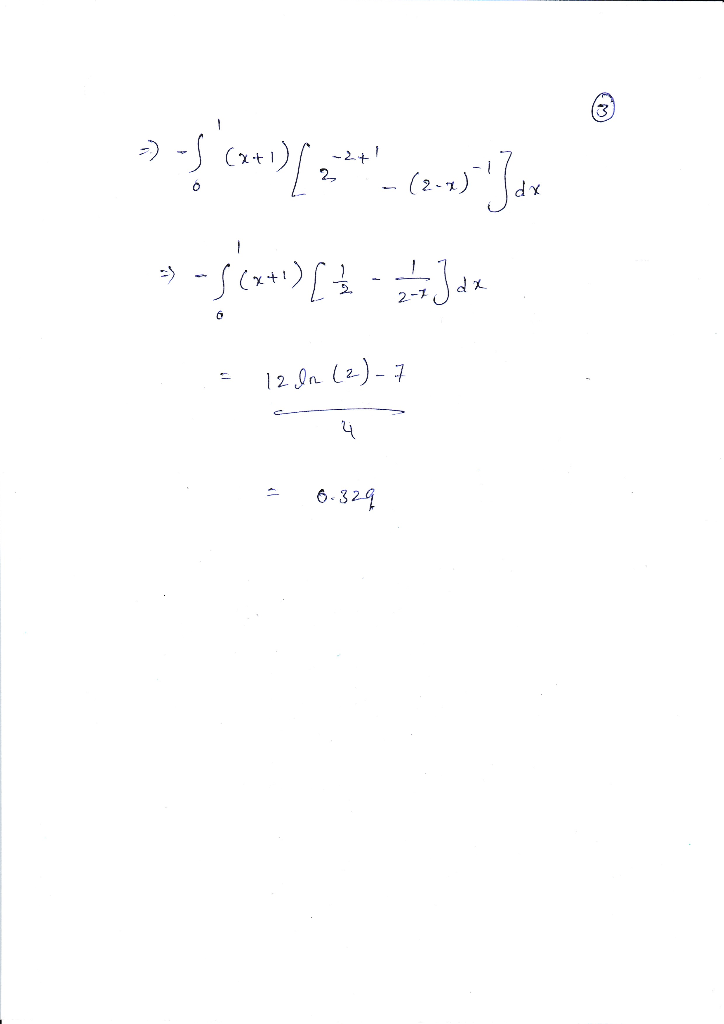#### Earn Coins

Coins can be redeemed for fabulous gifts.

Similar Homework Help Questions
• ### Let X and Y be continuous random variables with joint pdf f(x,y) =fX (c(X + Y),...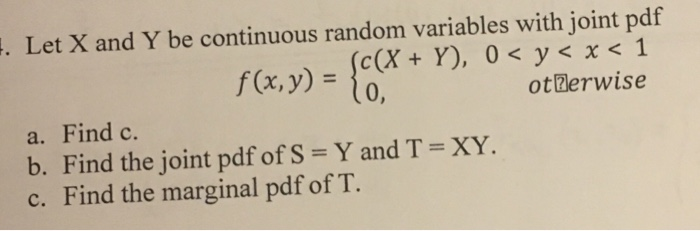Let X and Y be continuous random variables with joint pdf f(x,y) =fX (c(X + Y), 0 < y < x <1 otBerwise a. Find c. b. Find the joint pdf of S = Y and T = XY. c. Find the marginal pdf of T. 、

• ### Let X and Y be continuous random variables with joint pdf fx y (x, y)-3x, 0...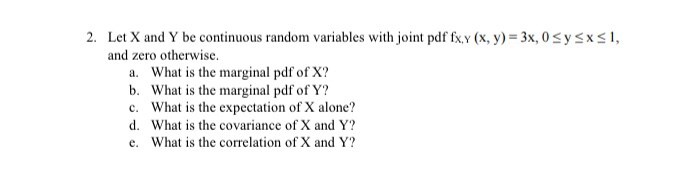Let X and Y be continuous random variables with joint pdf fx y (x, y)-3x, 0 Sy and zero otherwise. 2. sx, a. What is the marginal pdf of X? b. What is the marginal pdf of Y? c. What is the expectation of X alone? d. What is the covariance of X and Y? e. What is the correlation of X and Y?

• ### 7.5.6 Random variables X and Y have joint PDF fx,y(x, y) = _J1/2 -1 < x...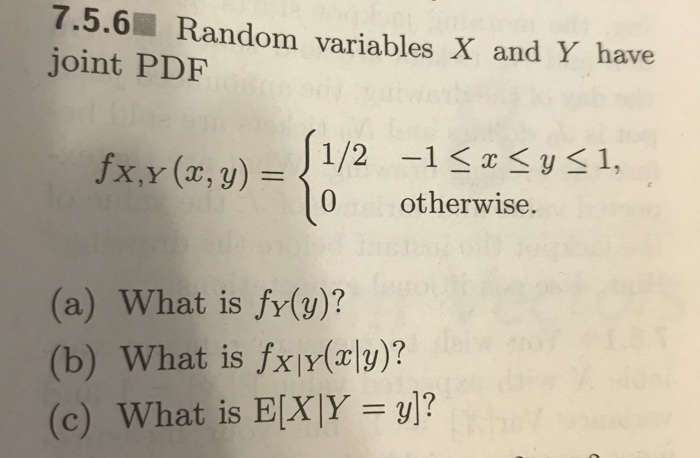7.5.6 Random variables X and Y have joint PDF fx,y(x, y) = _J1/2 -1 < x <y <1, 1/2 10 otherwise. (a) What is fy(y)? (b) What is fx|v(x\y)? (c) What is E[X|Y = y)?

• ### 4) The random variables X and Y have the joint PDF fx,y(x, y) = 0 <...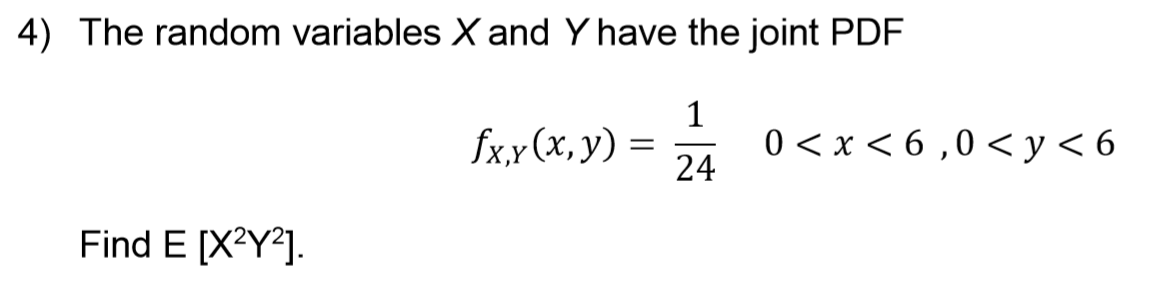4) The random variables X and Y have the joint PDF fx,y(x, y) = 0 < x < 6,0 < y < 6 Find E [X2Y2].

• ###  The joint probability density function of two continuous random variables X and Y is fx,x(x,...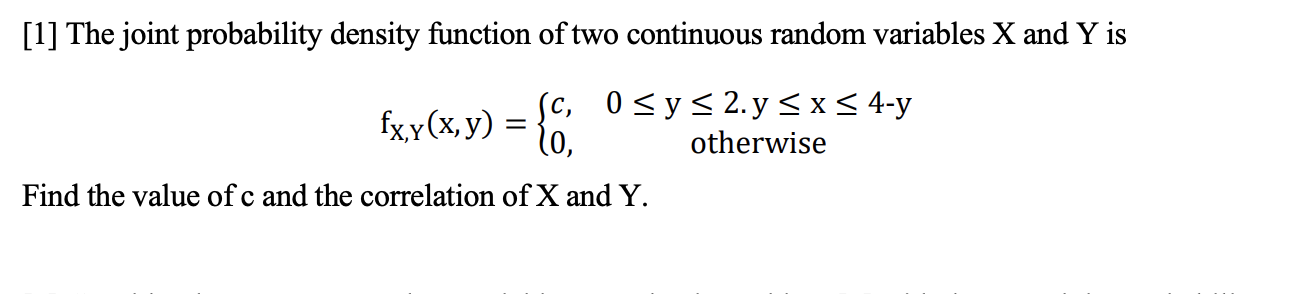The joint probability density function of two continuous random variables X and Y is fx,x(x, y) = {6. sc, 0 <y s 2.y = x < 4-y otherwise Find the value of c and the correlation of X and Y.

• ### Let X and Y be continuous random variables with joint pdf fx,r (x, y)-3x, 0Sysx3s1, and...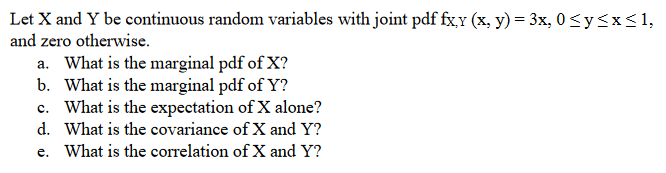Let X and Y be continuous random variables with joint pdf fx,r (x, y)-3x, 0Sysx3s1, and zero otherwise. a. What is the marginal pdf of X? b. What is the marginal pdf of Y? c. What is the expectation of X alone? d. What is the covariance of X and Y? e. What is the correlation of X and Y?

• ### Let X and Y be random variables with joint PDF fx,y(x, y) = 2 for 0...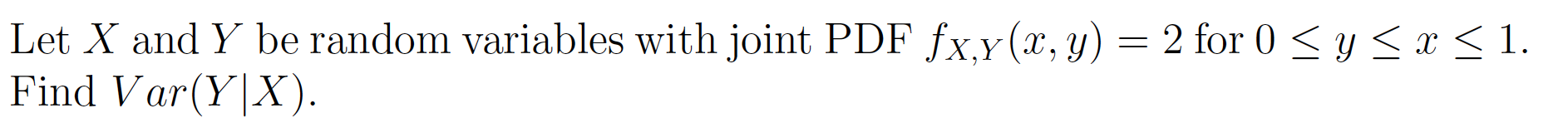Let X and Y be random variables with joint PDF fx,y(x, y) = 2 for 0 < y < x < 1. Find Var(Y|X).

• ### 1. Let X and Y be two jointly continuous random variables with joint CDF otherwsie a....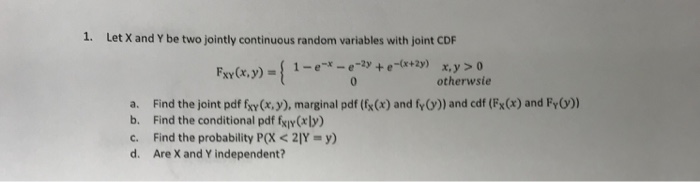1. Let X and Y be two jointly continuous random variables with joint CDF otherwsie a. Find the joint pdf fxy(x, y), marginal pdf (fx(x) and fy()) and cdf (Fx(x) and Fy)) b. Find the conditional pdf fxiy Cr ly c. Find the probability P(X < Y = y) d. Are X and Y independent?

• ### Consider the following joint PDF of continuous random variables X and Y: 22 – 2pxy +...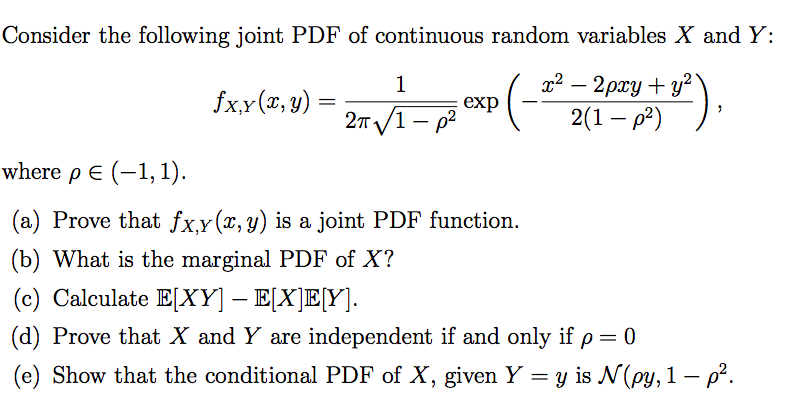Consider the following joint PDF of continuous random variables X and Y: 22 – 2pxy + y2 2(1 - 02) where pe(-1,1). (a) Prove that fx,y(x, y) is a joint PDF function. (b) What is the marginal PDF of X? (c) Calculate E[XY] – E[X]E[Y]. (d) Prove that X and Y are independent if and only if p= 0 (e) Show that the conditional PDF of X, given Y = y is N(py, 1 – p2.

• ### Question 3 [17 marks] The random variables X and Y are continuous, with joint pdf 0...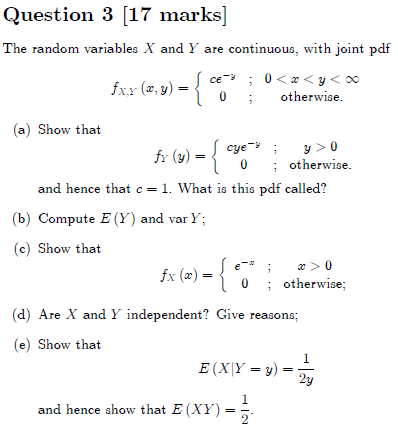Question 3 [17 marks] The random variables X and Y are continuous, with joint pdf 0 y otherwise ce fxx (,y) a) Show that cye fr (y) otherwise and hence that c = 1. What is this pdf called? (b) Compute E (Y) and var Y; (c) Show that { > 0 fx (a) e otherwise (d) Are X and Y independent? Give reasons; (e) Show that 1 E(XIY 2 and hence show that E (XY) =. Question 3 [17...